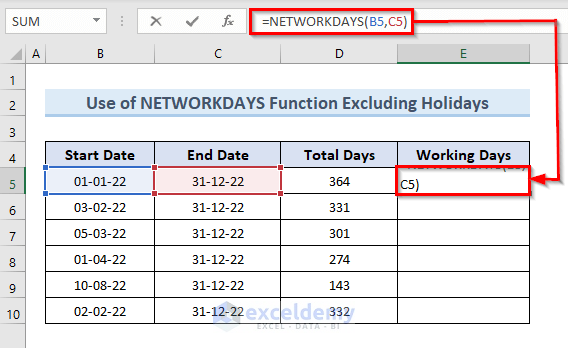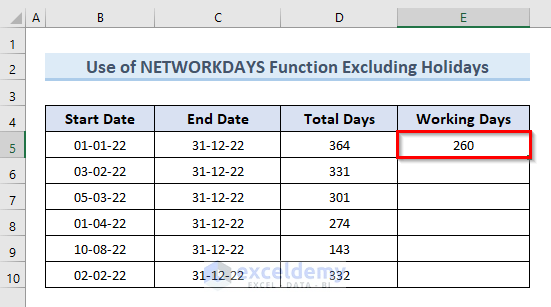# How to Calculate Working Days between Two Dates in Excel (4 Methods)

Get FREE Advanced Excel Exercises with Solutions!

In this tutorial, we will explain how to use different functions to calculate working days between two dates in Excel. Sometimes, it becomes very essential to calculate working days between two dates in project management. Throughout this article, we will use multiple functions with unique datasets to illustrate the process of calculating working days between two dates in Excel.

## How to Calculate Working Days between Two Dates in Excel: 4 Easy Methods

Microsoft Excel provides us with a lot of functions to perform different tasks. In the 4 methods of this article, we will use the NETWORKDAYS and the NETWORKDAYS.INTL functions are dedicated to calculating working days between two dates. We will also use a combination of the SUM, INT & WEEKDAY functions to get the same result.

### 1. Excel NETWORKDAYS Function to Calculate Working Days between Two Dates

Excel’s NETWORKDAYS function calculates the number of workdays between two dates. We can also exclude holidays from our working days which is an optional argument of this function.

#### 1.1 Calculate Working Days between Two Dates in Excel Excluding Holidays

In this method, we will use the NETWORKDAYS function to calculate working days between two dates. We will not consider the holidays in this method. So, this process will only consider Saturday and Sunday as weekends. In the following dataset, we have the Start Date and End Date for different works. We will calculate the total working days in that period in the column named Working Days.So, let’s see the steps to perform this action.

STEPS:

• Firstly, select cell E5. Insert the following formula in that cell:
`=NETWORKDAYS(B5,C5)`

####• Now, press Enter.
• The above action returns the value of working days between the dates “1-01-22” and “31-12-22” in cell E5. We can see from the following image that the value of working days for that range is 260.

####• Secondly, select cell E5. Move the mouse cursor to the bottom right corner of the selected cell so that it turns into a plus (+) sign like the following image.• Thirdly, click on the plus (+) sign and drag the Fill Handle down to cell E10 to copy the formula of cell E5 in other cells. We can also double-click on the plus (+) sign to get the same result.• After that, release the mouse click.
• Lastly, we can see the values of working days excluding holidays in cells (E5:E10).#### 1.2. Include Holidays While Calculating Working Days between Two Dates

Unlike the previous example, we will consider holidays in this example to calculate working days between two dates with the NETWORKDAYS function. To illustrate this method we will use the same dataset but this time we have an additional list of holidays. We can see the holidays in the following image of the dataset.Let’s take a look at the steps to perform this action.

STEPS:

• First, select cell E5. Input the following formula in that cell:
`=NETWORKDAYS(B6,C6,\$D\$13:\$D\$15)`• Now press Enter.
• The above command returns the number of working days between the dates “1-01-22” and “31-12-22”. The formula used in this method considers the value of the range (D13:D15) as a holiday. The value of working days for that range is 257, as seen in the following image.• Next, select cell E5. Drop the mouse pointer to the selected cell’s bottom right corner, where it will convert into a plus (+) sign, as shown in the image below.
• After that, to copy the formula of cell E5 in other cells click on the plus (+) sign and drag the Fill Handle down to cell E10. We can also double-click on the plus (+) sign to get the same result.• Now, free the mouse click.
• Finally, we get all the values of working days in cells (E5:E10).Read More: How to Find Last Business Day of Month in Excel

### 2. Apply NETWORKDAYS.INTL Function to Calculate Working Days with Custom Holidays

The NETWORKDAYS.INTL function is similar to the NETWORKDAYS function. Both of the functions calculate working days between two dates. But the NETWORKDAYS.INTL function allows us to select which day we will consider as a holiday. The NETWORKDAYS.INTL function considers only Sunday as an international holiday. So, it will consider Saturday as a working day. To illustrate this method we will perform the previous method again with the NETWORKDAYS.INTL function.So, just go through the following steps to perform this action.

STEPS:

• In the beginning, select cell E5. Write down the following formula in that cell:
`=NETWORKDAYS.INTL(B5,C5,11,\$D\$13:\$D\$15)`• Now, press Enter.
• The number of working days between the dates “1-01-22” and “31-12-22” is returned by the above command. This method’s calculation interprets the value of the range (D13:D15) as a holiday. we can see the number of working days in that range is 310. The value is larger than the previous results as it is not taking Saturday as a holiday.• Next, click on cell E5. Slide the mouse cursor to the bottom right corner of the selected cell, where it will turn into a plus (+) sign, as shown below.
• Then, click the plus (+) sign and drag the Fill Handle down to cell E10 to duplicate the formula from cell E5. To get the same outcome, we can double-click on the plus (+) sign.• After that, drop the mouse and click now.
• Finally, we have all of the working day’s values in cells (E5:E10).### 3. Calculate Number of Working Days in a Part-Time Job in Excel

The number of working days for a part-time job is not the same as the regular one. We have to add some extra modifications to the function NETWORKDAYS.INTL to extract the working days in a part-time job. To illustrate this method we will use the same dataset that we used in the first example.Let’s take a look at the steps to perform this method.

STEPS:

• Firstly, select cell E5. Insert the following formula in that cell:
`=NETWORKDAYS.INTL(B5,C5,"1010111")`• Now, hit Enter.
• The above command returns the number of part-time working dates between the dates “1-01-22” and “31-12-22“. We can see that the value of the working day between this range is 104.• Secondly, go to cell E5. Put the mouse cursor to the bottom right corner of the selected cell, where it will convert into a plus (+) sign, as shown in the figure below.
• Thirdly, tap on the plus (+) sign and drag the Fill Handle down to cell E10 to paste the formula from cell E5. We can double-click on the plus (+) sign to get the same result.• After that, free the mouse click.
• Lastly, we have all of the working day’s values in cells (E5:E10).NOTE:

In this method, we have used “1010111” in lieu of selecting the weekend from the function’s built-in option.

• 0 represents a working day.
• 1 represents a non-working day.

Here the first number of the sequence indicates Monday whereas the last number indicates Friday. So, the sequence “1010111” means that only Tuesday and Thursday are working days, and the rest of the days of the week are non-working days.

### 4. Combine Excel SUM and INT Functions to Calculate Working Days Between Two Dates

Apart from the NETWORKDAYS and the NETWORKDAYS.INTL function, there’s also another method that can be used to get the number of workdays between two dates. In this method, we will use a combination of the SUM and INT functions with the assistance of the WEEKDAY function to calculate working dates between two dates. In Excel, the SUM function is used to add multiple numbers together. The INT function converts a number to its closest integer value. The WEEKDAY function returns a weekday that matches a particular date. We’ll use the same dataset as in the first example to demonstrate this procedure.Now, just go through the steps to perform this method.

STEPS:

• First, select cell E5. Write down the following formula in that cell:
`=SUM(INT((WEEKDAY(B5-{2,3,4,5,6})+C5-B5)/7))`• Now, hit Enter.
• We get the value of working days between the dates “1-01-22” and “31-12-22” in cell E5 by the above command. We get the value 260 in cell E5.• Next, select cell E5. Move the mouse pointer to the bottom right corner of the selected cell, where it will switch into a plus (+) sign, as displayed below.
• Then, simply tap the plus (+) sign and drag the Fill Handle down to cell E10 to paste the formula from cell E5. To get the same outcome, we can double-click on the plus (+) sign also.• After that, release the mouse click.
• Finally, we get all the values of working days in cells (E5:E10).🔎 How Does the Formula Work?

• WEEKDAY(B5-{2,3,4,5,6}): The values 2,3,4,5 & 6 indicate five working days in a week starting from the date in cell B5.
• INT((WEEKDAY(B5-{2,3,4,5,6})+C5-B5)/7): This part returns a number of working days per week.
• SUM(INT((WEEKDAY(B5-{2,3,4,5,6})+C5-B5)/7)): Returns the total working days from date “1-01-22” to “31-12-22”.

## Conclusion

In conclusion, this post will show you how to calculate working days between two dates in Excel. To put your skills to the test, use the practice worksheet that comes with this article. Please leave a comment below if you have any questions. Our team will try to reply to you as soon as possible. In the future, keep an eye out for more unique Microsoft Excel solutions.

## Related ArticlesMukesh Dipto

Hi there! I am Mukesh Dipto. I graduated with a bachelor's degree in engineering. Currently, I am working as a technical content writer in ExcelDemy. You will find all my articles on Microsoft Excel on this site. Outside of the workplace, my hobbies and interests include sports and meeting new people. I also enjoy sports. My favorite sports are football (to watch and play) and Badminton (play).

1. ReplyHello, ExcelDemy 🙂

I kind of need your help on something …. unfortunately I couldn’t find anyone to know to resolve this & help me.

My problem is this one:

I have an excel with some activities, a gantt, and I want to write the formulas for the Progress column & also the formulas for the Estimated Days column (which represents the total days of woking) and Working Days (the actual working days on that moment), keeping in mind that I have completed manually the start column & the ddl column of the activities..

Is there any formula to help me calculate the dates from the 4 columns I told you about ?
Can you help me by letting me know the formulas & how to do it ?

Thank you so so much !
Irina

• ReplyMd. Abdur Rahim Rasel Jul 16, 2023 at 12:41 PM

Hello IRINA!
Thanks for sharing your problem with us!
It would be better for us if you shared your Excel dataset to help us better understand your problem.
Don’t worry! I have created an Excel file to fix your problem. I have solved your problem based on the current working days. I have calculated the Progress of the work & the Estimated Days of the work (which represent the total days of working), and Working Days (the actual working days at that moment).
Dates Calculation.xlsx
[email protected]
You can also leave your problem on our Forum site.
Regards
Md. Abdur Rahim Rasel
Exceldemy TeamAdvanced Excel Exercises with Solutions PDF